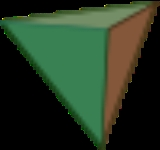xPlatonic solidOverview

In geometry
Geometry
Geometry arose as the field of knowledge dealing with spatial relationships. Geometry was one of the two fields of pre-modern mathematics, the other being the study of numbers ....

, a Platonic solid is a convex
Convex polytope
A convex polytope is a special case of a polytope, having the additional property that it is also a convex set of points in the n-dimensional space Rn...

polyhedron
Polyhedron
In elementary geometry a polyhedron is a geometric solid in three dimensions with flat faces and straight edges...

that is regular
Regular polyhedron
A regular polyhedron is a polyhedron whose faces are congruent regular polygons which are assembled in the same way around each vertex. A regular polyhedron is highly symmetrical, being all of edge-transitive, vertex-transitive and face-transitive - i.e. it is transitive on its flags...

, in the sense of a regular polygon
Regular polygon
A regular polygon is a polygon that is equiangular and equilateral . Regular polygons may be convex or star.-General properties:...

. Specifically, the faces of a Platonic solid are congruent
Congruence (geometry)
In geometry, two figures are congruent if they have the same shape and size. This means that either object can be repositioned so as to coincide precisely with the other object...

regular polygons, with the same number of faces meeting at each vertex
Vertex (geometry)
In geometry, a vertex is a special kind of point that describes the corners or intersections of geometric shapes.-Of an angle:...

; thus, all its edges are congruent, as are its vertices and angles.

There are five Platonic solids (shown below):

The name of each figure is derived from its number of faces: respectively 4, 6, 8, 12, and 20.

The aesthetic beauty
Mathematical beauty
Many mathematicians derive aesthetic pleasure from their work, and from mathematics in general. They express this pleasure by describing mathematics as beautiful. Sometimes mathematicians describe mathematics as an art form or, at a minimum, as a creative activity...

and symmetry
Symmetry
Symmetry generally conveys two primary meanings. The first is an imprecise sense of harmonious or aesthetically pleasing proportionality and balance; such that it reflects beauty or perfection...

of the Platonic solids have made them a favorite subject of geometers for thousands of years.